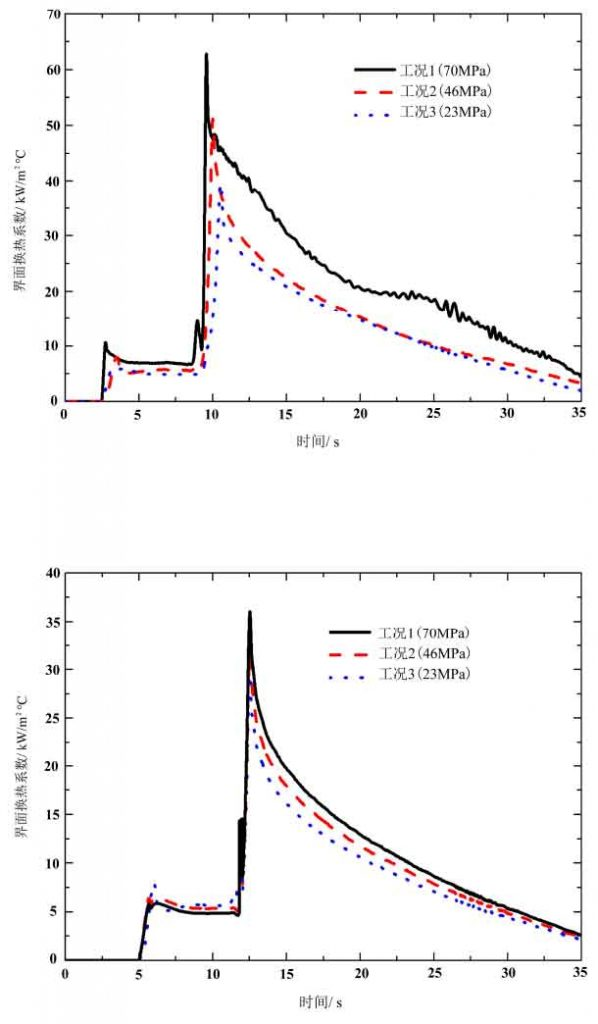# Effect of coating thickness on interfacial heat transfer coefficient in Squeeze Casting

The coating used was water-based graphite, and the coating thickness was measured by tt260b coating thickness gauge. In this paper, working conditions 1, 2 and 3 represent three working conditions with applied pressure of 70, 46 and 23 MPa. The initial mold temperature of condition 1 is 230 ℃, and the initial mold temperature of the other two conditions is 250 ℃, and the pouring temperature is 660 ℃.

As shown in Figure 1, before the application of the applied pressure, because the working conditions are basically the same, the variation law of the interface heat transfer coefficient is similar. At the beginning of pressure application, both the heat transfer coefficient and the interface pressure of the casting die interface increase abruptly and reach the maximum value. The larger the applied pressure is, the larger the maximum values of heat transfer coefficient, heat flux and pressure are. After the applied pressure, the interfacial heat transfer coefficient and pressure begin to decrease soon.(a) The coating thickness is 32 μ M (b) The coating thickness is 136 μ M

As shown in Figure 1 (a), when the coating thickness is 32 μ m, the interfacial heat transfer coefficient is very sensitive to the change of applied pressure. After the external pressure is applied, the interface heat transfer coefficient varies greatly under different external pressures. However, when the coating thickness is 136 μ m, as shown in Fig. 1 (b), the difference of interface heat transfer coefficient is very small under different applied pressure.

The roughness of die surface is about 25-50um. When the coating thickness is 32 μ m, the coating is mainly filled into the gap of the casting die interface, as shown in Fig. 3 (b). Because the coating is water-based graphite, the thermal conductivity is higher than that of air, so the interface thermal resistance is reduced and the interface heat transfer is strengthened. However, when the coating thickness is 136 μ m, a thick coating layer is formed on the casting die interface. As shown in Fig. 3 (c), the thermal resistance of the coating layer becomes the main factor affecting the interface heat transfer. The thicker the coating layer is, the greater the thermal resistance is.